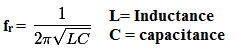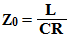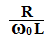## Series and Parallel Resonance

The Resonance condition of Series or Parallel AC Circuit has very important applications. There are some similarities and differences between Series Resonance and Parallel Resonance.
In this post, we will discuss the comparison between Series and Parallel Resonance and Applications.

### Series Resonance:

Here an RLC series circuit is shown. When the Inductive Reactance is equal to the Capacitive Reactance then the RLC Series circuit comes to the resonance condition.
For a certain value of applied Frequency, this condition arises and that frequency is called Resonant Frequency.
At the resonance condition, the inductive reactance and capacitance will be the same and they cancel each other.

### Parallel Resonance:

Here an RLC parallel circuit is shown. When the Net reactive or wattless component is equal to zero then the resonance occurs in the RLC parallel Circuit.
At resonance condition, the Net susceptance is equal to Zero.
At resonance condition,  the admittance is equal to the conductance.

### Comparison Between Series and Parallel Resonance:

#### Similarities:

(1) At Resonance Condition the Power Factor of the RLC Series Circuit is Unity.
At Resonance Condition, the power factor of the RLC parallel Circuit Also Unity(1).

(2) The equation of Resonant Frequency of RLC series Circuit is,The equation of Resonant Frequency of RLC Parallel Circuit is,(3) The Bandwidth of RLC series circuit at Resonance Condition,The Bandwidth of RLC parallel circuit at Resonance Condition,(4) The equation of Half  Power frequencies for RLC series circuit,The equation of Half  Power frequencies for RLC Parallel Circuit,#### Differences:

(1) At Resonance condition the Impedance of the RLC series circuit is Minimum.
But at Resonance condition the Impedance of the RLC Parallel Circuit is Maximum.

(2) At Resonance condition, the flow of current through the RLC Series Circuit will be Maximum.
But at Resonance condition, the flow of current through the RLC Parallel Circuit will be Minimum.

(3) At Resonance condition the Admittance of the RLC series circuit is Maximum.
But at Resonance condition the Admittance of the RLC Parallel Circuit is Minimum

(4) The equation of Effective Impedance for RLC series Circuit is,The equation of effective impedance for RLC parallel Circuit is,(5) The RLC series circuit amplifies Voltage at Resonance Condition.
The RLC parallel circuit amplifies Current at Resonance Condition.

(6) Q-Factor for Series Resonance,Q-Factor for Parallel Resonance,### Applications of Series Resonance Circuit:

(1) The main application of Series Resonance circuit is Tuning. They are used for Tuning purpose.
(2) Series Resonance circuit also used as Oscillator Circuit.
(3) Series resonance circuit is used as a Voltage Amplifier.
(4) Series Resonance circuits are used in the communication system for signal processing.
(5) Series resonance Circuit also used as High-frequency filter circuit.

### Application of Parallel Resonance Circuit:

(1) The parallel resonance circuit is also used for Tuning purpose.
(2) The parallel resonance circuit is used in Induction heating system.
(3) The parallel resonance circuit is used as a Current Amplifier.
(4) The parallel resonance circuit is also used as a filter circuit.
(5) The parallel resonance circuits are used in RF amplifiers.

Thank you for visiting the website. keep visiting for more updates.

RLC Series and Parallel RESONANCE Comparison and ApplicationsReviewed by Author on March 06, 2019 Rating: 5